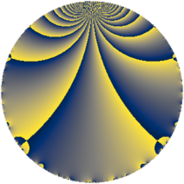# Properties

 Label 57.2.fLevel $57$ Weight $2$ Character orbit 57.f Rep. character $\chi_{57}(8,\cdot)$ Character field $\Q(\zeta_{6})$ Dimension $8$ Newform subspaces $1$ Sturm bound $13$ Trace bound $0$

# Related objects

## Defining parameters

 Level: $$N$$ $$=$$ $$57 = 3 \cdot 19$$ Weight: $$k$$ $$=$$ $$2$$ Character orbit: $$[\chi]$$ $$=$$ 57.f (of order $$6$$ and degree $$2$$) Character conductor: $$\operatorname{cond}(\chi)$$ $$=$$ $$57$$ Character field: $$\Q(\zeta_{6})$$ Newform subspaces: $$1$$ Sturm bound: $$13$$ Trace bound: $$0$$

## Dimensions

The following table gives the dimensions of various subspaces of $$M_{2}(57, [\chi])$$.

Total New Old
Modular forms 16 16 0
Cusp forms 8 8 0
Eisenstein series 8 8 0

## Trace form

 $$8 q + 4 q^{6} - 16 q^{7} - 4 q^{9} + O(q^{10})$$ $$8 q + 4 q^{6} - 16 q^{7} - 4 q^{9} - 12 q^{10} + 12 q^{13} + 4 q^{16} - 12 q^{21} + 12 q^{22} + 4 q^{24} - 12 q^{25} + 12 q^{28} - 8 q^{30} + 24 q^{33} + 24 q^{34} + 16 q^{39} - 12 q^{40} - 20 q^{42} - 16 q^{43} + 32 q^{45} + 24 q^{48} - 48 q^{51} - 24 q^{52} - 4 q^{54} + 16 q^{55} - 28 q^{57} - 64 q^{58} - 24 q^{60} + 28 q^{61} + 8 q^{63} - 32 q^{64} - 4 q^{66} + 36 q^{70} - 24 q^{72} + 12 q^{73} + 60 q^{76} + 36 q^{78} + 24 q^{79} + 28 q^{81} + 16 q^{82} + 32 q^{87} + 12 q^{90} - 48 q^{91} - 4 q^{93} + 72 q^{96} + 24 q^{97} - 32 q^{99} + O(q^{100})$$

## Decomposition of $$S_{2}^{\mathrm{new}}(57, [\chi])$$ into newform subspaces

Label Dim $A$ Field CM Traces $q$-expansion
$a_{2}$ $a_{3}$ $a_{5}$ $a_{7}$
57.2.f.a $8$ $0.455$ $$\Q(\zeta_{24})$$ None $$0$$ $$0$$ $$0$$ $$-16$$ $$q+(-\zeta_{24}+\zeta_{24}^{5}+\zeta_{24}^{7})q^{2}+(\zeta_{24}^{2}+\cdots)q^{3}+\cdots$$